Compound Interest

Chapter 8 Class 8 Comparing Quantities
Concept wise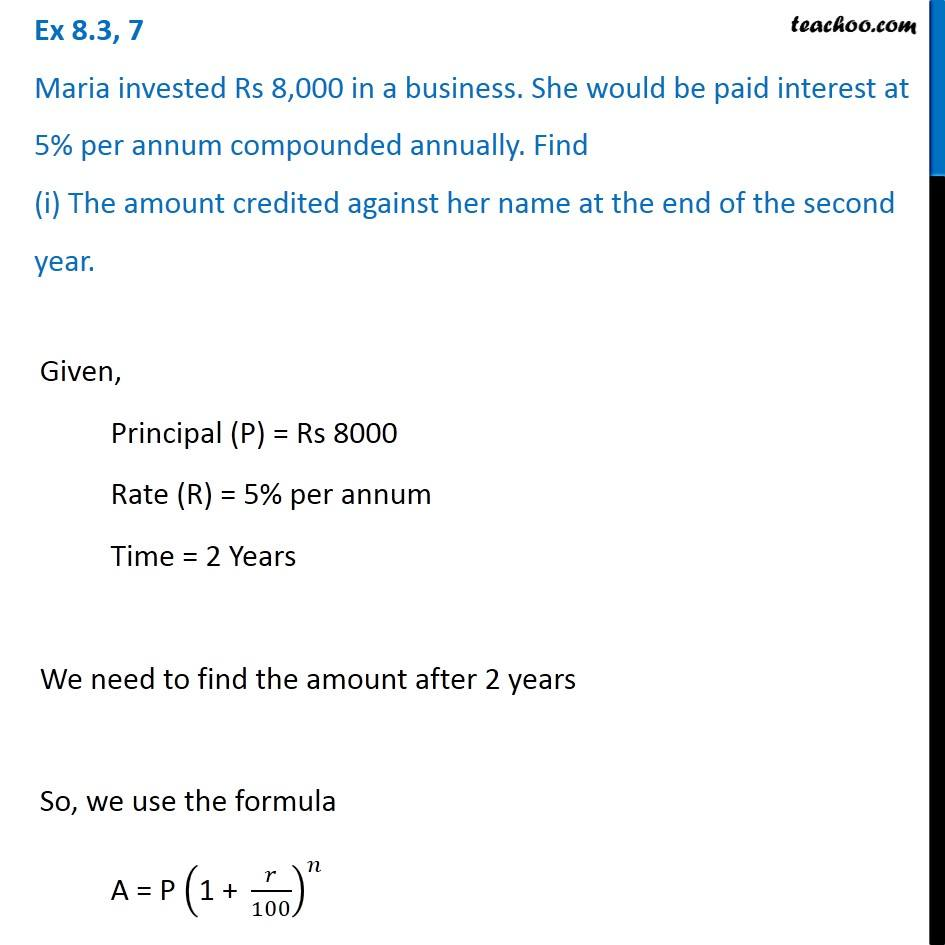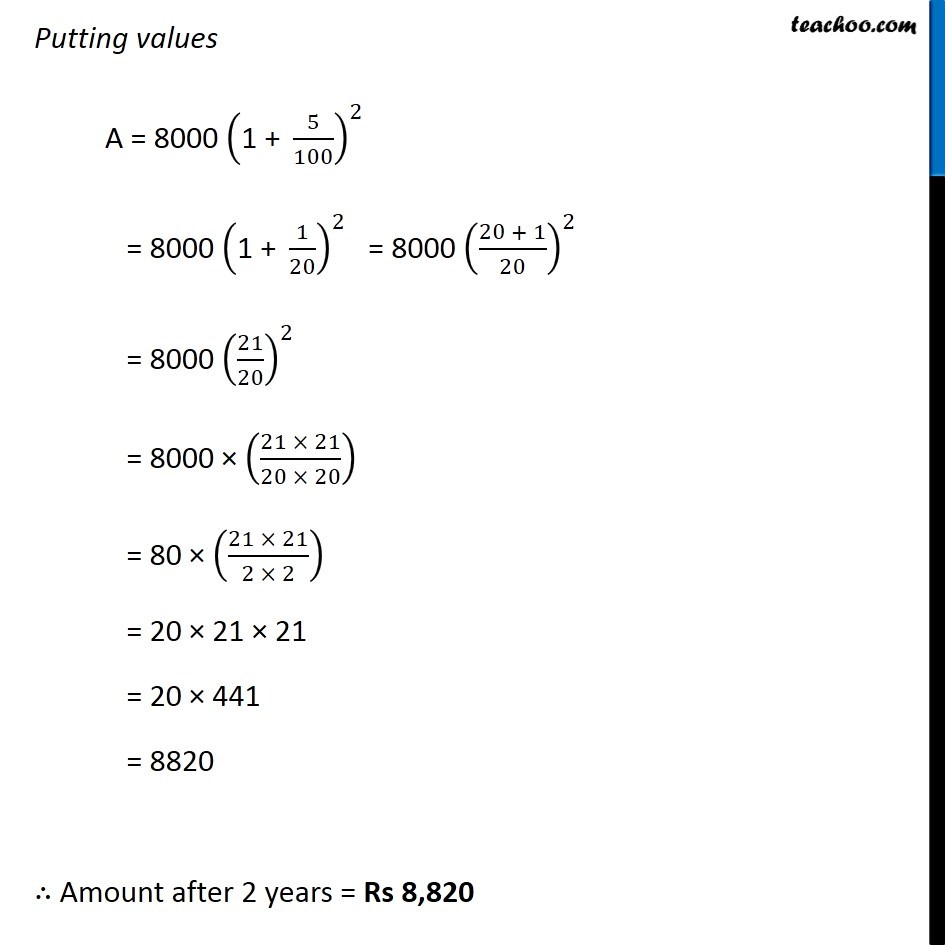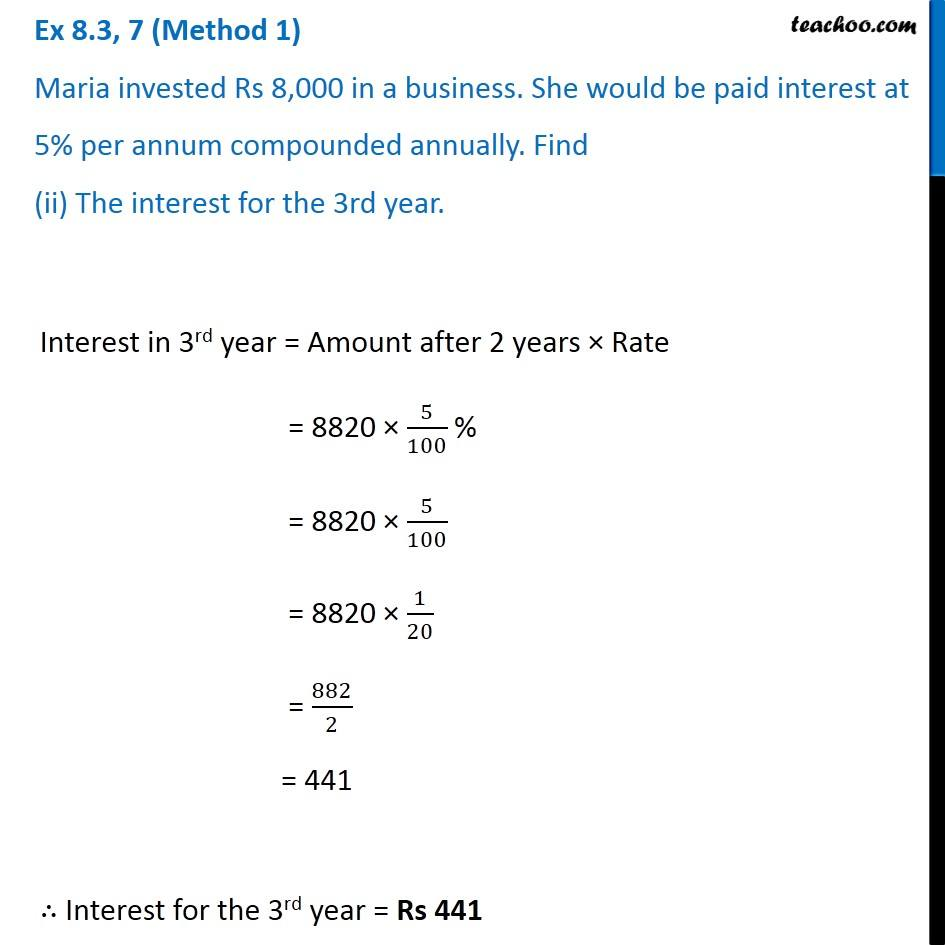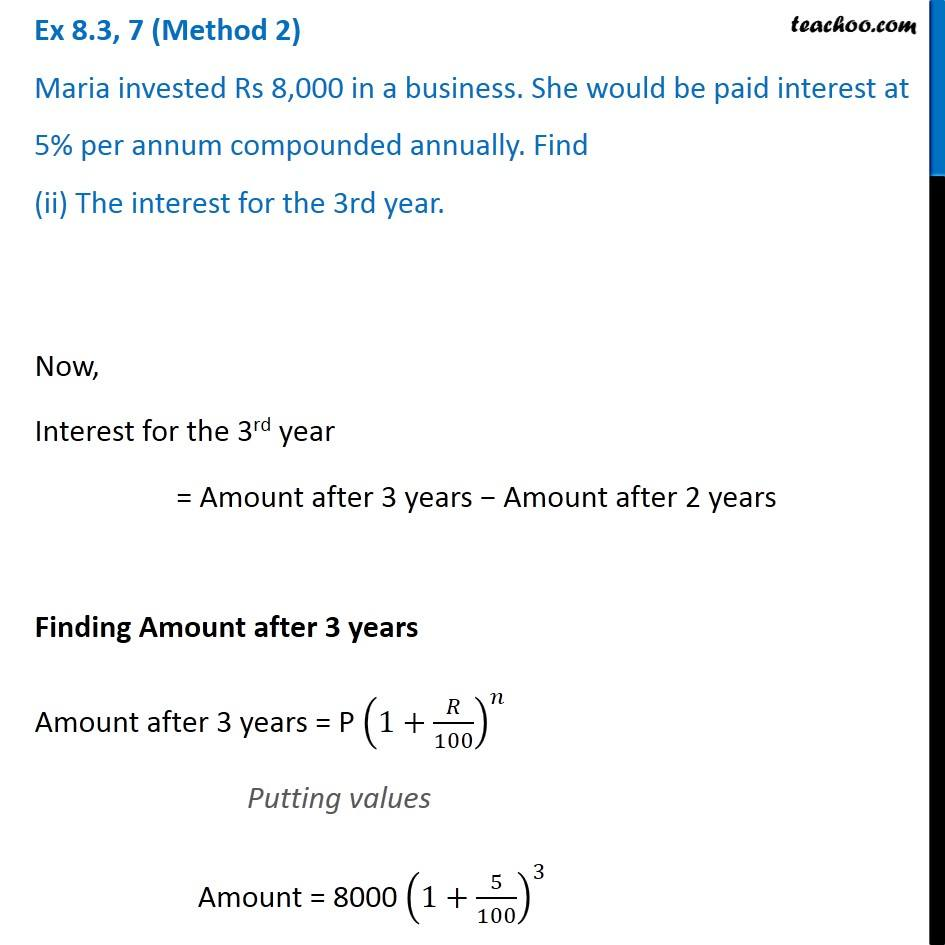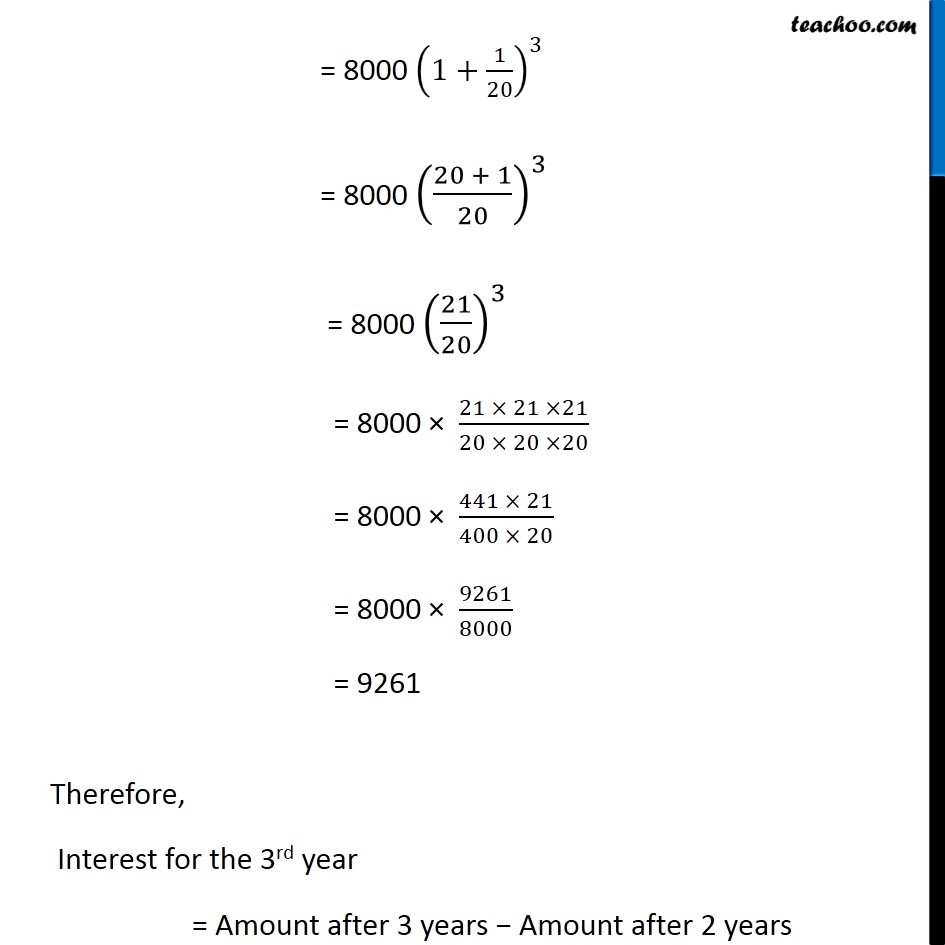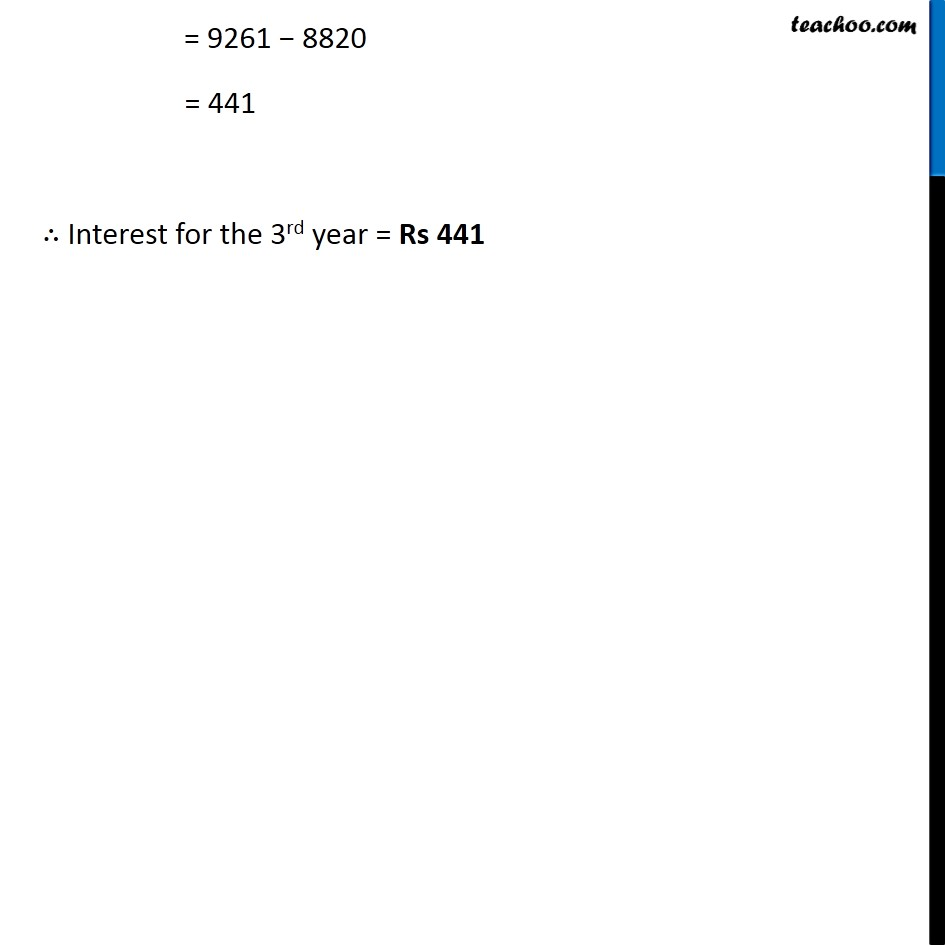Introducing your new favourite teacher - Teachoo Black, at only ₹83 per month

### Transcript

Ex 8.3, 7 Maria invested Rs 8,000 in a business. She would be paid interest at 5% per annum compounded annually. Find (i) The amount credited against her name at the end of the second year. Given, Principal (P) = Rs 8000 Rate (R) = 5% per annum Time = 2 Years We need to find the amount after 2 years So, we use the formula A = P ("1 + " 𝑟/100)^𝑛 Putting values A = 8000 ("1 + " 5/100)^2 = 8000 ("1 + " 1/20)^2 = 8000 ((20 + 1)/20)^2 = 8000 (21/20)^2 = 8000 × ((21 × 21)/(20 × 20)) = 80 × ((21 × 21)/(2 × 2)) = 20 × 21 × 21 = 20 × 441 = 8820 ∴ Amount after 2 years = Rs 8,820 Ex 8.3, 7 (Method 1) Maria invested Rs 8,000 in a business. She would be paid interest at 5% per annum compounded annually. Find (ii) The interest for the 3rd year. Interest in 3rd year = Amount after 2 years × Rate = 8820 × 5/100 % = 8820 × 5/100 = 8820 × 1/20 = 882/2 = 441 ∴ Interest for the 3rd year = Rs 441 Ex 8.3, 7 (Method 2) Maria invested Rs 8,000 in a business. She would be paid interest at 5% per annum compounded annually. Find (ii) The interest for the 3rd year. Now, Interest for the 3rd year = Amount after 3 years − Amount after 2 years Finding Amount after 3 years Amount after 3 years = P (1+𝑅/100)^𝑛 Putting values Amount = 8000 (1+5/100)^3 = 8000 (1+1/20)^3 = 8000 ((20 + 1)/20)^3 = 8000 (21/20)^3 = 8000 × (21 × 21 ×21)/(20 × 20 ×20) = 8000 × (441 × 21)/(400 × 20) = 8000 × 9261/8000 = 9261 Therefore, Interest for the 3rd year = Amount after 3 years − Amount after 2 years = 9261 − 8820 = 441 ∴ Interest for the 3rd year = Rs 441